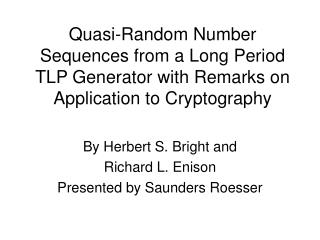DownloadDownload PresentationBy Herbert S. Bright and Richard L. Enison Presented by Saunders Roesser

# By Herbert S. Bright and Richard L. Enison Presented by Saunders Roesser

Download Presentation## By Herbert S. Bright and Richard L. Enison Presented by Saunders Roesser

- - - - - - - - - - - - - - - - - - - - - - - - - - - E N D - - - - - - - - - - - - - - - - - - - - - - - - - - -
##### Presentation Transcript

1. Quasi-Random Number Sequences from a Long Period TLP Generator with Remarks on Application to Cryptography By Herbert S. Bright and Richard L. Enison Presented by Saunders Roesser

2. The Problem • Generation of successful random number sequences that pass all statistical testing criteria. • Generation in an Application domain.

3. Background • Physical Generations are unsuitable for modern computers • Linear Congruential formulas: • Xi+1 = axi + c (mod m) • Additive Formulas • Xi = a1Xx-1 + a2xi-2+…..+apxi-p+ c (mod m) • Don’t work unless you have large primes.

4. TLP Sequence • Tausworthe-Lewis-Payne distribution • Sequence for generation of random numbers. • Trinomial: x521+x32+1 • Generate 64-bit numbers • Period is 2521-1 • Better then linear congruential generators

5. Statistical Testing Criteria • Equidistribution/Frequency Test • The number of time a given number falls into a given interval • Serial Test • The number of times a sequence appears in a certain number of numbers • Gap Test • The distribution of gaps in the sequence of various lengths.

6. More Tests • Runs Test • Plots the distribution of maximal ascending runs of various lengths • Coupon Collector’s Test • Choose a small interger, divide the number into intervals then plot the distribution runs of various lengths required to have all intervals represented

7. More Tests • Permutation Test • Order relations between the members of the sequence in groups of k. • Serial Correlation Test • Computer the correlation coefficient between consecutive members of the sequence. • Others..

8. Results • At the time, all present generators failed the battery of tests. • Hope came from recursive function theory. • TLP Generator showed good results in string tests • Passed equidistributivity tests, along with other tests.

9. Other Physical Random Number Generators • Dice • Ionizing radiation • Gas discharge tubes • Leaky capacitors • Physical noise generators